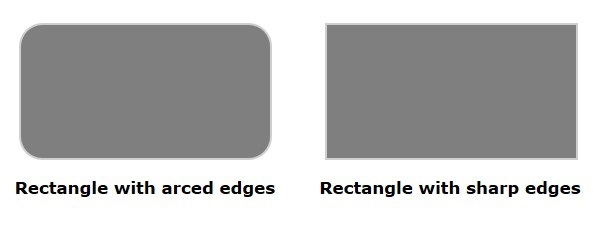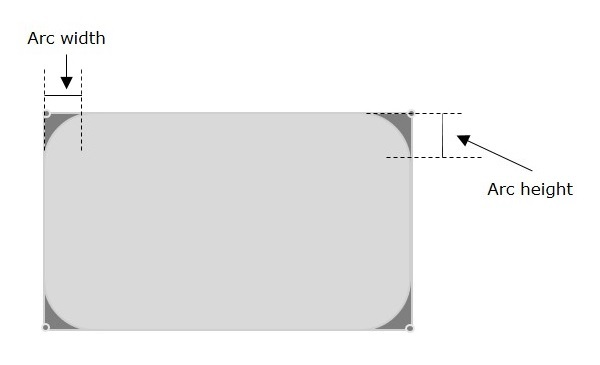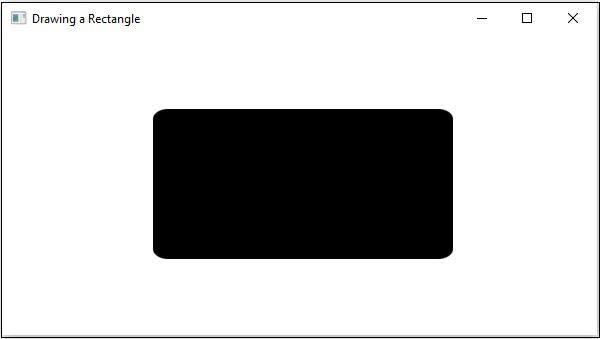# JavaFX - 2D Shapes Rounded Rectangle

In JavaFX, you can draw a rectangle either with sharp edges or with arched edges as shown in the following diagram.The one with arched edges is known as a rounded rectangle and it has two additional properties namely −

• arcHeight − The vertical diameter of the arc, at the corners of a rounded rectangle.

• arcWidth − The horizontal diameter of the arc at the corners of a rounded rectangle.By default, JavaFX creates a rectangle with sharp edges unless you set the height and width of the arc to +ve values (0<) using their respective setter methods setArcHeight() and setArcWidth().

## Example

Following is a program which generates a rounded rectangle using JavaFX. Save this code in a file with the name RoundedRectangle.java.

```import javafx.application.Application;
import javafx.scene.Group;
import javafx.scene.Scene;
import javafx.stage.Stage;
import javafx.scene.shape.Rectangle;

public class RoundedRectangle extends Application {
@Override
public void start(Stage stage) {
//Drawing a Rectangle
Rectangle rectangle = new Rectangle();

//Setting the properties of the rectangle
rectangle.setX(150.0f);
rectangle.setY(75.0f);
rectangle.setWidth(300.0f);
rectangle.setHeight(150.0f);

//Setting the height and width of the arc
rectangle.setArcWidth(30.0);
rectangle.setArcHeight(20.0);

//Creating a Group object
Group root = new Group(rectangle);

//Creating a scene object
Scene scene = new Scene(root, 600, 300);

//Setting title to the Stage
stage.setTitle("Drawing a Rectangle");

stage.setScene(scene);

//Displaying the contents of the stage
stage.show();
}
public static void main(String args[]){
launch(args);
}
}
```

Compile and execute the saved java file from the command prompt using the following commands.

```javac RoundedRectangle.java
java RoundedRectangle
```

On executing, the above program generates a JavaFX window displaying a rounded rectangle as shown below.javafx_2d_shapes.htm